MHD boundary layer flow due to a moving wedge in a parallel stream with the induced magnetic field

Article metrics

• 3953 Accesses

• 7 Citations

Abstract

The present analysis considers the steady magnetohydrodynamic (MHD) laminar boundary layer flow of an incompressible electrically conducting fluid caused by a continuous moving wedge in a parallel free stream with a variable induced magnetic field parallel to the wedge walls outside the boundary layer. Using a similarity transformation, the governing system of partial differential equations is first transformed into a system of ordinary differential equations in the form of a two-point boundary value problem (BVP) and then solved numerically using a finite difference scheme known as the Keller box method. Numerical results are obtained for the velocity profiles and the skin friction coefficient for various values of the moving parameter λ, the wedge parameter β, the reciprocal magnetic Prandtl number α and the magnetic parameter S. Results indicate that when the wedge and the fluid move in the opposite directions, multiple solutions exist up to a critical value $λ c$ of the moving parameter λ, whose value depends on the values of S and β.

MSC: 34B15, 76D10.

1 Introduction

Magnetohydrodynamics (MHD) is a subject that studies the behavior of an electrically conducting fluid in the presence of an electromagnetic field with applications in many different fields of engineering as well as geophysics, astrophysics, manufacturing, etc. The subject of MHD has been applied, for example, in problems associated with the confinement of plasma by magnetic fields and in projects involving thermonuclear generation of energy. In recent years it has been widely used in metallurgy industries involving sheet-like materials such as production of paper, polymer sheets and wire drawing and in horizontal continuous casting of hollow billets. For examples of these applications, see Li et al.  and Yan et al. . Historically, the study of the hydrodynamic behavior of the boundary layer on a semi-infinite flat plate in the presence of a uniform transverse magnetic field has been first considered by Rossow . Since then, the study of MHD flow and heat transfer fields past moving surfaces has drawn considerable attention with variations in types of geometrical surfaces and types of fluids.

The steady laminar flow of a viscous and incompressible fluid passing a fixed wedge was first analyzed in the early 1930s by Falkner and Skan  to illustrate the application of Prandtl’s boundary layer theory, in which a similarity transformation was used to reduce the boundary layer equations to an ordinary differential equation known as the Falkner-Skan equation. The Falkner-Skan equation also represents the boundary layer flow with stream-wise pressure gradient. The general cases with $β=0$ were numerically studied by Fang  and Weidman et al.  independently. There are many references on the solutions of Falkner-Skan equations; for example, see Hartree , Hastings , Brodie and Banks , Pantokratoras , Alizadeh et al. , Yao , and Abbasbandy and Hayat . Similarity solutions for pressure gradient driven flow over a stretching boundary were analyzed by Riley and Weidman  for the case of external velocity and boundary velocity being proportional to the same powers of the downstream coordinate. Very interesting and extensive results were reported demonstrating a rich variety of solutions available, including the existence of multiple solutions, and an exact solution was also presented for $β=−1$. Fang and Zhang  studied a special case of the Falkner-Skan equation with $β=−1$ in the presence of wall suction and injection. An exact solution was presented for the boundary conditions with both wall mass transfer and wall movement, with different solution behavior identified in different solution regions. On the other hand, Ishak et al.  considered the steady MHD boundary layer flow in a conducting fluid flowing transverse to a variable magnetic field along a moving wedge in a free stream. The results reported were consistent with those found by Riley and Weidman  and with earlier studies by the same authors Ishak et al. [17, 18]. More recent studies on similar problems were done by Van Gorder and Vajravelu , Postelnicu and Pop  and Parand et al. .

The present work aims to study the boundary layer flow over a moving wedge in a parallel free stream of an electrically conducting fluid with the induced magnetic field. It considers an extension of the results reported by Riley and Weidman  and Ishak et al.  on the flow characteristics of a moving wedge in a parallel free stream. Both studies reported the existence of multiple solutions when the fluid and the wedge move in the opposite directions within a specific range of moving parameter λ and a critical value $λ c$ beyond which the solution is non-existent. The present study considers the corresponding MHD flow of the paper by Ishak et al. , but with the induced magnetic field, and investigates how this magnetic field affects the flow and the critical value $λ c$. The induced magnetic field is assumed to be applied parallel to the wedge walls at the outer edge of the boundary layer. Such an induced magnetic field has been also considered by Davies , Apelblat [23, 24], Kumari et al. , Takhar et al.  and more recently by Kumari and Nath . To obtain the solutions, the governing partial differential equations are first transformed into ordinary differential equations using a similarity transformation. The ordinary differential equations obtained are then solved numerically by a very efficient finite difference scheme known as the Keller box method for some values of the selected parameters. The effect of the induced magnetic field on the flow field for different values of the wedge parameter β is included in the analysis. Particular cases of the present results are compared with those reported by Riley and Weidman  and Ishak et al. [16, 17].

2 Basic equations

Consider the steady laminar flow of an incompressible electrically conducting fluid caused by a continuous moving wedge in a parallel free stream with a variable induced magnetic field applied parallel to the wedge walls outside the boundary layer (inviscid flow). Following Apelblat  or Cowling , the basic equations for the flow of a viscous, electrically conducting, incompressible fluid can be written in a vectorial form as follows: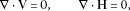(1)(2)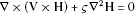(3)

where V is the fluid velocity vector, H is the induced magnetic field vector, $P=(p+μ | H | 2 /8π)$ is the magneto-hydrodynamic pressure, p is the fluid pressure, μ, ν, σ, ρ and $ς= ( 4 π μ σ ) − 1$ denote the magnetic permeability, kinematic viscosity, electric conductivity, fluid density and magnetic diffusivity, respectively. We take the Cartesian coordinates x measured along the surface of the wedge and y normal to it, respectively. If $(u,v)$ and $( H 1 , H 2 )$ are the velocity and magnetic components in $(x,y)$ directions, respectively, subject to the boundary layer approximations, equations (1)-(3) for the problem under consideration can be reduced to(4)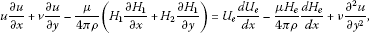(5)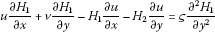(6)

where $U e (x)$ and $H e (x)$ are the x-velocity and magnetic field at the edge of the boundary layer, respectively. We assume here that $U e (x)= U ∞ x m$ and $H e (x)= H 0 x m$, where $U ∞$ is the constant velocity at the outer edge of the boundary layer and $H 0$ is the value of $H e (x)$ at $x=0$. Further, m is also a constant, which varies in the range $0≤m≤1$.

We will take the boundary conditions of equations (4)-(6) to be

(7)

where $U w$ is a positive or a negative constant. By applying the similarity variables

$ψ= [ 2 ν x U e ( x ) ( m + 1 ) ] 1 / 2 f(η), H 1 = H 0 x m g ′ (η),η= [ ( m + 1 ) U e ( x ) 2 ν x ] 1 / 2 y,$
(8)

equations (4)-(6) can be reduced to the following system of nonlinear ordinary differential equations: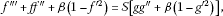(9)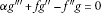(10)

subject to the boundary conditions (7) which are now transformed to

$f ( 0 ) = 0 , f ′ ( 0 ) = λ , f ′ ( ∞ ) = 1 , g ( 0 ) = 0 , g ′ ( 0 ) = 0 , g ′ ( ∞ ) = 1$
(11)

where primes denote differentiation with respect to η. Further, λ is the moving parameter, α is the reciprocal magnetic Prandtl number, β is the wedge parameter and S, the ratio of the magnetic to dynamic pressure, is the magnetic parameter. These parameters are defined as

$λ= U w U ∞ ,α= 1 4 π ν μ σ ,β= 2 m m + 1 ,S= μ H 0 2 / 8 π ρ U ∞ 2 / 2 .$
(12)

We notice that different values of β characterize a number of main-stream flows. For $β=0$, equations (9) and (10) are reduced to the MHD Blasius problem. The values $β=1$ and $0<β<1$ are equivalent to the flow past a wedge placed symmetrically in a stream. For MHD boundary layers, we take the values of the parameters S and α to be in the range $S≤1$ and $α≥1$; see Davies  and Kumari et al. . This is the same range of magnetic parameter adopted by Takhar et al.  and several earlier researchers investigating similar problems. It is also consistent with the existence of the steady-state solution of the ‘super Alfven’ flow.

The physical quantity of interest is the skin friction coefficient which is defined as

$C f = τ w ρ U e 2 ,$
(13)

where the wall shear stress is given by $τ w =μ ( ∂ u / ∂ y ) y = 0$. Using the similarity variables (8), we obtain

$Re x 1 / 2 C f = [ 2 ( 1 + m ) ] 1 / 2 f ″ (0)$
(14)

where $Re x = U e (x)x/ν$ is the local Reynolds number.

We also notice that for $λ=0$ the present problem corresponds to the MHD boundary layer flow over a static wedge, which has been considered by Apelblat , in which the MHD wedge problem was solved using the Laplace transform method to give an infinite series approximation solution for $f ″ (0)$ and $g ″ (0)$. On the other hand, it may be noted that for $S=0$ (without a magnetic field), equation (9) reduces to that of Ishak et al. . Therefore, as $S=0$ implies the absence of a magnetic field, equation (10) governing the induced magnetic field is no longer necessary.

3 Results and discussion

Nonlinear ordinary differential equations (9) and (10) subject to the boundary conditions (11) form a two-point boundary value problem (BVP) and are solved numerically using the Keller box method as described in the book by Cebeci and Bradshaw . In this method, the solution is obtained using the following four steps:

1. (i)

Reduce equations (9) and (10) to a first-order system.

2. (ii)

Write the difference equations using centered differences.

3. (iii)

Linearize the resulting algebraic equations by Newton’s method and write them in the matrix-vector form.

4. (iv)

Solve the linear systems by the block-tridiagonal-elimination technique.

The numerical method is then programmed using MATLAB R2010a software. To obtain a numerical solution, it is required to make an appropriate guess for the step size of η, Δη and the thickness of the boundary layer $η ∞$ (typically a finite number between 4 to 10 is chosen). Beginning with some initial guess value of $η ∞$, equations (9) and (10) subject to the boundary conditions (11) together with some particular set of parameters are solved to obtain the velocity profiles $f ′ (η)$ and the induced magnetic profiles $g ′ (η)$. The solution process is repeated until further changes (increment) in $η ∞$ do not lead to any changes in the values of $f ″ (0)$ and $g ″ (0)$ or, in other words, the results are independent of the value of $η ∞$. The initial step size employed is $h=Δη=0.01$. The skin friction coefficient $f ″ (0)$, the velocity profiles $f ′ (η)$, the induced magnetic profiles $g ′ (η)$ and the rate of change of the induced magnetic field, which we will henceforth call the induced magnetic gradient, $g ″ (0)$ are obtained for various values of the governing parameters, namely the moving parameter λ, the wedge parameter β and the magnetic parameter S. In order to assess the accuracy of the numerical method used, we have compared some of our results for the non-magnetic case ($S=0$) with those obtained by Riley and Weidman , Rajagopal et al. , Ishak et al.  and Kuo . Table 1 presents values of the skin friction coefficient $f ″ (0)$ for $λ=0$ and various values of the wedge parameter β for the non-magnetic case ($S=0$). Table 2 compares the values of the skin friction $f ″ (0)$ for the set of triple solutions computed when $λ=−0.4$, $β=0.05$ with those obtained by Riley and Weidman . We observed that the results obtained for the present study are found to be in very good agreement with those obtained by earlier researchers. Therefore, the developed code can be used with confidence for the magnetic case ($0).

Variations of the velocity profiles $f ′ (η)$ and the induced magnetic profiles $g ′ (η)$ with the moving parameter λ, the wedge parameter β, the magnetic parameter S and the reciprocal magnetic Prandtl number α are presented in Figures 1 to 4. All the sample profiles satisfy the far field boundary conditions (11) asymptotically, thus supporting the numerical results obtained. From these figures, we see that as the values of λ and β increase, both the fluid velocity $f ′ (η)$ and the induced magnetic field $g ′ (η)$ also increase while the velocity boundary layer thickness decreases. In contrast, as the values of S and α increase, the fluid velocity and the induced magnetic decrease while the velocity boundary layer thickness increases. We also notice that the effect of the reciprocal magnetic Prandtl number α is more pronounced on $g ′ (η)$ compared to $f ′ (η)$.

Figure 5 presents the variation of the skin friction coefficient $f ″ (0)$ as a function of λ for various values of S when the wedge parameter and reciprocal magnetic Prandtl number are fixed at $β=0.5$ and $α=1$, respectively. It was found that for all values of the magnetic parameter S, with $0≤S<1$, the solution is unique for all values of $λ≥ λ c$, where $λ c$ is the minimum value of λ for which the solution exists. The critical value $| λ c |$ decreases as the value of S increases. In our calculation for the case $β=0.5$, the solution stops to exist when the value of the induced magnetic gradient reaches $g ″ (0)=0$. As is evident from Figure 5, the value of the skin friction coefficient $f ″ (0)$ also decreases as the value of the magnetic parameter S increases. Furthermore, this decrease becomes more rapid for higher values of S.

Figure 6 presents the variation of the skin friction coefficient $f ″ (0)$ and the induced magnetic gradient $g ″ (0)$ as a function of λ for various values of the magnetic parameter S when the wedge parameter is fixed at $β=0.7$. The figure indicates that for all values of S ($0≤S<1$), the solution is unique for all values of $λ≥0$, while dual solutions exist for some range of values of $λ c ≤λ<0$. Furthermore, the skin friction coefficient $f ″ (0)$, the induced magnetic gradient $g ″ (0)$ and the critical value $| λ c |$ decrease as the value of S increases. As evident from Table 3, the critical value $λ c$ for $β=0$ and $β=1$ in the non-magnetic case ($S=0$) that we computed in this study shows an excellent agreement with previously reported result by Klemp and Acrivos  and Hussaini et al. . The case of $β=0$ corresponds to the flat plate, while $β=1$ refers to the stagnation point flow.

Figure 7 shows the velocity profiles $f ′ (η)$ at the critical values of λ ($= λ c$), prior to separation, for various values of S when $β=0.7$. We observe that as the value of S increases, the critical value $| λ c |$, the induced magnetic gradient $g ″ (0)$ and the skin friction $f ″ (0)$ decrease, thus supporting our previous observation from Figure 6. We also notice that the induced magnetic gradient $g ″ (0)$ varies almost linearly with the moving parameter λ, with most of the second solution having a very small value of $| g ″ (0)|< 10 − 4$. Figure 8 shows velocity $f ′ (η)$ and induced magnetic field $g ′ (η)$ profiles supporting the existence of a dual solution when $β=0.7$, $S=0.3$ and $λ=−0.85$.

Figure 9 illustrates the variation of the skin friction coefficient $f ″ (0)$ and the induced magnetic gradient $g ″ (0)$ as a function of λ for various values of the magnetic parameter S when the wedge parameter is fixed at $β=0.03$. The figure indicates that for all values of S ($0≤S<1$), the solution is unique for all values of $λ≥0$, while triple solutions exist for some range of values of $λ c ≤λ<0$. Similar to the previous considered cases, the skin friction coefficient $f ″ (0)$, the induced magnetic gradient $g ″ (0)$ and the critical value $| λ c |$ also decrease as the value of S increases. Here, we also found that for the second and third solutions, the values of the induced magnetic gradient are also usually very small $| g ″ (0)< 10 − 4 |$.

Figure 10 presents the variation of the skin friction coefficient $f ″ (0)$ as a function of λ for various values of the wedge parameter β when the magnetic parameter is fixed at $S=0.3$. Here the value of the reciprocal magnetic Prandtl number is also fixed at $α=1$. The result exhibits similar characteristics as those obtained in the non-magnetic case ($S=0$) reported by Riley and Weidman . We can see that the value of $f ″ (0)$ increases as β increases, and there is a critical value $λ c$ of the moving parameter beyond which the similarity solutions do not exist. The magnitude of the critical value $| λ c |$ also increases as the wedge parameter β increases. Figure 7 also indicates a rich variety of solutions depending on the value of the wedge parameter β. Following Riley and Weidman  for the non-magnetic case ($S=0$), we draw particular attention to the following interesting features of the solution set in the presence of the magnetic field with $S=0.3$. For $0.6<β≤1$, there is a unique solution for $λ≥0$ and dual solutions for some range of $λ c <λ<0$; for $0.1<β≤0.6$, the solution is unique for all $λ≥ λ c$; for $0≤β≤0.1$, triple solutions are available for some range of values of the parameter λ. To be more specific, our computation shows that for $β=0.03$, a unique solution has been found for the range $−0.8365≤λ≤−0.2502$ and $λ≥−0.336$, while triple solutions have been found for the range $−0.335≤λ≤−0.2503$. This result is qualitatively consistent with the result reported by Riley and Weidman , where triple solutions were found for $0<β<0.14$, a unique solution for all λ when $0.14<β<0.5$ and dual solutions for some range of λ when $0.5<β<1$. We mention here that Riley and Weidman  reported that for the non-magnetic case ($S=0$), all solution curves for $β>0$ have the point $(1,0)$ as a limit point. In the present study, our computation shows that the solution curves terminate near the point $(−0.8365,0)$, as it is evident from Figure 7. Riley and Weidman  explained the significance of this limit point in terms of the ‘edge’ $η e$ of the boundary layer. Further, the value of $η e$ increases as $f ″ (0)$ decreases, until the limit point is approached, $η e =∞$. We expect this limit point to move further right if the value of the parameter S is increased. Figures 11, 12, 13 present samples of velocity profiles and induced magnetic profiles supporting the existence of triple solutions for $β=0$, $β=0.03$ and $β=0.1$, respectively.

Figure 14 shows the variation of the induced magnetic gradient $g ″ (0)$ as a function of the moving parameter λ with the wedge parameter β. We observe that the induced magnetic gradient $g ″ (0)$ increases as the wedge parameter β increases for smaller values of λ but varies very little with β for larger values of λ.

Following the convention adopted by earlier researchers, we define the first two upper branches of solutions as those for which $f ″ (0)$ is greater for a given value of β, while the third branch is that with the smallest value of $f ″ (0)$. We notice that the velocity profiles $f ′ (η)$ for the first two upper branches of solutions exhibit the same monotonic behavior. The boundary layer for the first branch is usually very thin and the velocity profile $f ′ (η)$ rapidly attains the value $f ′ (∞)=1$. In general, the third branch of solutions usually involves a much larger boundary layer thickness compared to the other two branches. It is usually characterized by starting off with a rather small value of $f ″ (0)>0$, with a non-monotonic behavior in the development of the velocity profiles $f ′ (η)$, before assuming its final asymptotic value $f ′ (∞)=1$. Similar non-monotonic behavior was reported by Riley and Weidman  when they considered the velocity profiles $f ′ (η)$ of the upper branch solution for $−1≤β<−0.5$. Following Ishak et al. , we postulate that the upper branch of solutions with the highest value of $f ″ (0)$ (first solutions) are physically stable and occur in practice since it is the only solution for $λ>0$, i.e., when the fluid and the solid surface move in the same direction.

A reduction in the skin friction $f ″ (0)$ implies a reduction in the drag force. Thus, the magnetic field reduces the drag force and speeds up the separation. On the other hand, increasing the included angle of the wedge will increase the drag force, hence delaying the separation. This result is consistent with that reported by Ishak et al. .

According to the Lorenz law, the induced magnetic field will oppose the change in the original magnetic field rather than the field itself. If, for example, the original field is decreasing, then the induced magnetic field must be in the same direction as the original field to oppose the decrease. From Figures 6 and 9, we see that the induced magnetic gradient $g ″ (0)$ increases monotonically with the increasing value of λ. This increase is supposedly opposing a decrease in the original magnetic field. Furthermore, the induced magnetic gradient also decreases with the increase in S, which is consistent with the Lorenz law. We also notice that the effect of both S and β is more pronounced on the skin friction $f ″ (0)$ compared to the induced magnetic gradient $g ″ (0)$.

4 Conclusions

In this paper, we have considered similarity solutions for the steady MHD boundary layer flow due to a continuous moving wedge in a parallel free stream with the induced magnetic field. We investigated the effects of the moving parameter λ, the ratio of magnetic to dynamic pressure S, the wedge parameter β and the reciprocal magnetic Prandtl number α on the flow field and the induced magnetic field characteristics. It has been found that increasing the values of the moving parameter λ and the wedge parameter β speeds up the fluid flow. In contrast, increasing the ratio of magnetic to dynamic pressure S and the reciprocal magnetic Prandtl number α slows down the fluid flow. Furthermore, the skin friction or the surface shear stress $f ″ (0)$ and the induced magnetic gradient $g ″ (0)$ decrease with the increase of the ratio of magnetic to dynamic pressure S, but increase with the wedge parameter β. We have also demonstrated the existence of a rich variety of solutions by varying the value of the wedge parameter β. We have also found that when the wedge and the fluid move in the same direction, the solution is unique for all values of the parameters β and S. However, when the wedge and the free stream move in the opposite directions, multiple solutions exist for some range of values of the moving parameter λ as soon as the value of the moving parameter is greater than a critical value $λ= λ c$. This critical value of λ is dependent on both parameters β and S. It has been found that increasing the wedge parameter β will increase the value of $| λ c |$, while increasing the ratio of magnetic to dynamic pressure S will reduce it. Thus, increasing the ratio of magnetic to dynamic pressure speeds up the boundary layer separation, while increasing the wedge parameter β delays it.

References

1. 1.

Li X, Guo Z, Zhou X, Wei B, Chen F, Ting B: Continuous casting of copper tube billets under rotating electromagnetic field. Mater. Sci. Eng. 2007, 460-461: 648-651.

2. 2.

Yan Z, Li X, Qi ZC, Zhang X, Li T: Study on horizontal electromagnetic continuous casting of CuNi10Fe1Mn alloy hollow billets. Mater. Des. 2009, 30: 2072-2076. 10.1016/j.matdes.2008.08.047

3. 3.

Rossow, VJ: On flow of electrically conducting fluid over a flat plate in the presence of a magnetic field. NACA TR. 1358 (1958)

4. 4.

Falkner VM, Skan SW: Some approximate solutions of the boundary-layer equations. Philos. Mag. 1931, 12: 865-896.

5. 5.

Fang T: Further study on a moving-wall boundary-layer problem with mass transfer. Acta Mech. 2003, 163: 183-188. 10.1007/s00707-002-0979-9

6. 6.

Weidman PD, Kubitschek DG, Davis AMJ: The effect of transpiration on self-similar boundary layer flow over moving surfaces. Int. J. Eng. Sci. 2006, 44: 730-737. 10.1016/j.ijengsci.2006.04.005

7. 7.

Hartree DR: On an equation occurring in Falkner and Skan’s approximate treatment of the equations of the boundary layer. Proc. Camb. Philos. Soc. 1937, 33: 223-239. 10.1017/S0305004100019575

8. 8.

Hastings SP: Reversed flow solutions of the Falkner-Skan equation. SIAM J. Appl. Math. 1972, 22: 329-334. 10.1137/0122031

9. 9.

Brodie P, Banks WHH: Further properties of the Falkner-Skan equation. Acta Mech. 1986, 65: 205-211.

10. 10.

Pantokratoras A: The Falkner-Skan flow with constant wall temperature and variable viscosity. Int. J. Therm. Sci. 2006, 45: 378-389. 10.1016/j.ijthermalsci.2005.06.004

11. 11.

Alizadeh E, Farhadi M, Sedeghi K, Ebrahim-Kerbia HR, Ghoafourian A: Solution of the Falkner-Skan equation for wedge by Adomian decomposition method. Commun. Nonlinear Sci. Numer. Simul. 2009, 14: 724-733. 10.1016/j.cnsns.2007.11.002

12. 12.

Yao B: Approximate analytical solution to the Falkner-Skan wedge flow with the permeable wall of uniform suction. Commun. Nonlinear Sci. Numer. Simul. 2009, 14: 3320-3326. 10.1016/j.cnsns.2009.01.014

13. 13.

Abbasbandy S, Hayat T: Solution of the MHD Falkner-Skan flow by homotopy analysis method. Commun. Nonlinear Sci. Numer. Simul. 2009, 14: 3591-3598. 10.1016/j.cnsns.2009.01.030

14. 14.

Riley N, Weidman PD: Multiple solutions of the Falkner-Skan equation for flow past a stretching boundary. SIAM J. Appl. Math. 1989, 49: 1350-1358. 10.1137/0149081

15. 15.

Fang T, Zhang J: An exact analytical solution of the Falkner-Skan equation with mass transfer and wall stretching. Int. J. Non-Linear Mech. 2008, 43: 1000-1006. 10.1016/j.ijnonlinmec.2008.05.006

16. 16.

Ishak A, Nazar R, Pop I: MHD boundary layer flow past a moving wedge. Magnetohydrodynamics 2009, 45: 3-10.

17. 17.

Ishak A, Nazar R, Pop I: Moving wedge and flat plate in a micropolar fluid. Int. J. Eng. Sci. 2006, 44: 1225-1236. 10.1016/j.ijengsci.2006.08.005

18. 18.

Ishak A, Nazar R, Pop I: Falkner-Skan equation for flow past a moving wedge with suction or injection. J. Appl. Math. Comput. 2007, 25: 67-83. 10.1007/BF02832339

19. 19.

Van Gorder RA, Vajravelu K: Existence and uniqueness results for a nonlinear differential equation arising in MHD Falkner-Skan flow. Commun. Nonlinear Sci. Numer. Simul. 2010, 15: 2272-2277. 10.1016/j.cnsns.2009.09.014

20. 20.

Postelnicu A, Pop I: Falkner-Skan boundary layer flow of a power-law fluid past a stretching wedge. Appl. Math. Comput. 2011, 217: 4359-4368. 10.1016/j.amc.2010.09.037

21. 21.

Parand K, Rezaei AR, Ghaderi SM: A approximate solution of the MHD Falkner-Skan flow by Hermite functions pseudospectral method. Commun. Nonlinear Sci. Numer. Simul. 2011, 16: 274-283. 10.1016/j.cnsns.2010.03.022

22. 22.

Davies TV: The magneto-hydrodynamic boundary layer in the two-dimensional steady flow past a semi-infinite flat plate I. Uniform conditions at infinity. Proc. R. Soc. Lond. Ser. A, Math. Phys. Sci. 1963, 273: 496-508. 10.1098/rspa.1963.0105

23. 23.

Apelblat A: Application of the Laplace transformation to the solution of the boundary layer equations. II magneto-hydrodynamic Blasius problem. J. Phys. Soc. Jpn. 1968, 25: 888-891. 10.1143/JPSJ.25.888

24. 24.

Apelblat A: Applications of the Laplace transform to the solution of the boundary layer equations. III magnetohydrodynamic Falkner-Skan problem. J. Phys. Soc. Jpn. 1969, 27: 235-239. 10.1143/JPSJ.27.235

25. 25.

Kumari M, Takhar HS, Nath G: MHD flow and heat transfer over a stretching surface with prescribed wall temperature or heat flux. Wärme-Stoffübertrag. 1990, 25: 331-336.

26. 26.

Takhar HS, Chamka AJ, Nath G: Unsteady flow and heat transfer on a semi-infinite flat plate with aligned magnetic field. Int. J. Eng. Sci. 1999, 37: 1723-1736. 10.1016/S0020-7225(98)00144-X

27. 27.

Kumari M, Nath G: Steady mixed convection stagnation-point flow of upper convected Maxwell fluids with magnetic field. Int. J. Non-Linear Mech. 2009, 44: 1048-1055. 10.1016/j.ijnonlinmec.2009.08.002

28. 28.

Cowling TG: Magnetohydrodynamics. Interscience, New York; 1957.

29. 29.

Cebeci T, Bradshaw P: Physical and Computational Aspects of Convective Heat Transfer. Springer, New York; 1988.

30. 30.

Rajagopal KR, Gupta AS, Nath TY: A note on the Falkner-Skan flows of a non-Newtonian fluid. Int. J. Non-Linear Mech. 1983, 18: 313-320. 10.1016/0020-7462(83)90028-8

31. 31.

Kuo BL: Application of the differential transformation method to the solutions of Falkner-Skan wedge flow. Acta Mech. 2003, 164: 161-174. 10.1007/s00707-003-0019-4

32. 32.

Klemp JB, Acrivos AA: A method for integrating the boundary-layer equations through a region of reverse flow. J. Fluid Mech. 1972, 53: 177-199. 10.1017/S0022112072000096

33. 33.

Hussaini MY, Lakin WD, Nachman A: On similarity solutions for laminar boundary layer problem with an upstream moving wall. SIAM J. Appl. Math. 1987, 47: 699-709. 10.1137/0147048

Acknowledgements

The authors gratefully acknowledge the financial support received in the form of a FRGS research grant from the Ministry of Higher Education, Malaysia, and DIP-2012-31 from the Universiti Kebangsaan, Malaysia. They also wish to express their sincere thanks to the reviewers for the valuable comments and suggestions.

Author information

Correspondence to Khamisah Jafar.

Competing interests

The authors declare that they have no competing interests.

Authors’ contributions

The paper is the result of joint work of all authors who contributed equally to the final version of the paper. All authors read and approved the final manuscript.

Authors’ original submitted files for images

Below are the links to the authors’ original submitted files for images.

Rights and permissions

Open Access This article is distributed under the terms of the Creative Commons Attribution 2.0 International License (https://creativecommons.org/licenses/by/2.0), which permits unrestricted use, distribution, and reproduction in any medium, provided the original work is properly cited.

Reprints and Permissions